# Electric Potential Energy: Potential Difference Notes | Study Basic Physics for IIT JAM - Physics

## Physics: Electric Potential Energy: Potential Difference Notes | Study Basic Physics for IIT JAM - Physics

The document Electric Potential Energy: Potential Difference Notes | Study Basic Physics for IIT JAM - Physics is a part of the Physics Course Basic Physics for IIT JAM.
All you need of Physics at this link: Physics

Automated external defibrillator unit (AED)Introduction:

In Electric Charge and Electric Field, we just scratched the surface (or at least rubbed it) of electrical phenomena. Two of the most familiar aspects of electricity are its energy and voltage. We know, for example, that great amounts of electrical energy can be stored in batteries, are transmitted cross-country through power lines, and may jump from clouds to explode the sap of trees. In a similar manner, at molecular levels, ions cross cell membranes and transfer information. We also know about voltages associated with electricity. Batteries are typically a few volts, the outlets in your home produce 120 volts, and power lines can be as high as hundreds of thousands of volts. But energy and voltage are not the same thing. A motorcycle battery, for example, is small and would not be very successful in replacing the much larger car battery, yet each has the same voltage. In this chapter, we shall examine the relationship between voltage and electrical energy and begin to explore some of the many applications of electricity.

Electric Potential Energy: Potential Difference

When a free positive charge q is accelerated by an electric field, it is given kinetic energy. The process is analogous to an object being accelerated by a gravitational field. It is as if the charge is going down an electrical hill where its electric potential energy is converted to kinetic energy. Let us explore the work done on a charge q by the electric field in this process, so that we may develop a definition of electric potential energy.
A charge accelerated by an electric field is analogous to a mass going down a hill. In both cases potential energy is converted to another form. Work is done by a force, but since this force is conservative, we can write W = -ΔPE.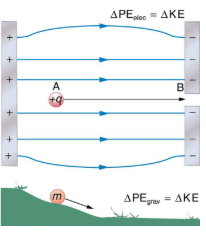The electrostatic or Coulomb force is conservative, which means that the work done on q is independent of the path taken. This is exactly analogous to the gravitational force in the absence of dissipative forces such as friction. When a force is conservative, it is possible to define a potential energy associated with the force, and it is usually easier to deal with the potential energy (because it depends only on position) than to calculate the work directly.
We use the letters PE to denote electric potential energy, which has units of joules (J). The change in potential energy, PE, is crucial, since the work done by a conservative force is the negative of the change in potential energy; that is, W=-PE. For example, work W done to accelerate a positive charge from rest is positive and results from a loss in PE, or a negative PE. There must be a minus sign in front of PE to make W positive. PE can be found at any point by taking one point as a reference and calculating the work needed to move a charge to the other point.

Potential Energy:

W=-PE. For example, work W done to accelerate a positive charge from rest is positive and results from a loss in PE, or a negative PE. There must be a minus sign in front of PE to make W positive. PE can be found at any point by taking one point as a reference and calculating the work needed to move a charge to the other point.
Gravitational potential energy and electric potential energy are quite analogous. Potential energy accounts for work done by a conservative force and gives added insight regarding energy and energy transformation without the necessity of dealing with the force directly. It is much more common, for example, to use the concept of voltage (related to electric potential energy) than to deal with the Coulomb force directly.
Calculating the work directly is generally difficult, since W = Fd cosθ and the direction and magnitude of F can be complex for multiple charges, for odd-shaped objects, and along arbitrary paths. But we do know that, since F = qE, the work, and hence PE, is proportional to the test charge q. To have a physical quantity that is independent of test charge, we define electric potential V (or simply potential, since electric is understood) to be the potential energy per unit charge: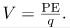Electric Potential:
This is the electric potential energy per unit charge.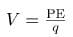Since PE is proportional to q, the dependence on q cancels. Thus V does not depend on q. The change in potential energy PE is crucial, and so we are concerned with the difference in potential or potential difference ΔV between two points, where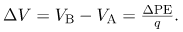The potential difference between points A and B, VB-VA, is thus defined to be the change in potential energy of a chargemoved from A to B, divided by the charge. Units of potential difference are joules per coulomb, given the name volt (V) after Alessandro Volta.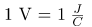Potential Difference:

The potential difference between points A and B VB-VA, is defined to be the change in potential energy of a charge q moved from A to B, divided by the charge. Units of potential difference are joules per coulomb, given the name volt (V) after Alessandro Volta.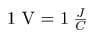The familiar term voltage is the common name for potential difference. Keep in mind that whenever a voltage is quoted, it is understood to be the potential difference between two points. For example, every battery has two terminals, and its voltage is the potential difference between them. More fundamentally, the point you choose to be zero volts is arbitrary. This is analogous to the fact that gravitational potential energy has an arbitrary zero, such as sea level or perhaps a lecture hall floor.
In summary, the relationship between potential difference (or voltage) and electrical potential energy is given by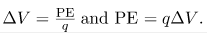Potential Difference and Electrical Potential Energy:
The relationship between potential difference (or voltage) and electrical potential energy is given by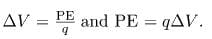The second equation is equivalent to the first.

Voltage is not the same as energy. Voltage is the energy per unit charge. Thus a motorcycle battery and a car battery can both have the same voltage (more precisely, the same potential difference between battery terminals), yet one stores much more energy than the other since PE = qΔV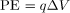. The car battery can move more charge than the motorcycle battery, although both are 12 V batteries.

Calculating Energy
Suppose you have a 12.0 V motorcycle battery that can move 5000 C of charge, and a 12.0 V car battery that can move 60,000 C of charge. How much energy does each deliver? (Assume that the numerical value of each charge is accurate to three significant figures.)

Strategy

To say we have a 12.0 V battery means that its terminals have a 12.0 V potential difference. When such a battery moves charge, it puts the charge through a potential difference of 12.0 V, and the charge is given a change in potential energy equal to PE=qΔV
So to find the energy output, we multiply the charge moved by the potential difference.

Solution

For the motorcycle battery, q=5000 C and ΔV=12.0. The total energy delivered by the motorcycle battery is

Similarly, for the car battery, q= 60,000 C and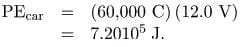Discussion

While voltage and energy are related, they are not the same thing. The voltages of the batteries are identical, but the energy supplied by each is quite different. Note also that as a battery is discharged, some of its energy is used internally and its terminal voltage drops, such as when headlights dim because of a low car battery. The energy supplied by the battery is still calculated as in this example, but not all of the energy is available for external use.

Note that the energies calculated in the previous example are absolute values. The change in potential energy for the battery is negative, since it loses energy. These batteries, like many electrical systems, actually move negative charge—electrons in particular. The batteries repel electrons from their negative terminals (A) through whatever circuitry is involved and attract them to their positive terminals (B). The change in potential is ΔV=VB-VA=+12V and the charge q is negative, so that  PE = qΔV is negative, meaning the potential energy of the battery has decreased when q has moved from A to B.
A battery moves negative charge from its negative terminal through a headlight to its positive terminal. Appropriate combinations of chemicals in the battery separate charges so that the negative terminal has an excess of negative charge, which is repelled by it and attracted to the excess positive charge on the other terminal. In terms of potential, the positive terminal is at a higher voltage than the negative. Inside the battery, both positive and negative charges move.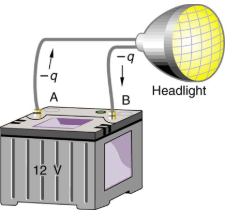How Many Electrons Move through a Headlight Each Second?
When a 12.0 V car battery runs a single 30.0 W headlight, how many electrons pass through it each second?

Strategy

To find the number of electrons, we must first find the charge that moved in 1.00 s. The charge moved is related to voltage and energy through the equation PE=qΔV. A 30.0 W lamp uses 30.0 joules per second. Since the battery loses energy, we have PE=-30.0 J and, since the electrons are going from the negative terminal to the positive, we see that

ΔV = +12.0V.

Solution

To find the charge q moved, we solve the equation PE = qΔV: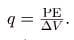Entering the values for PE and V, we get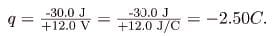The number of electrons ne is the total charge divided by the charge per electron. That is,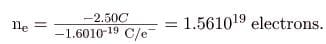Discussion

This is a very large number. It is no wonder that we do not ordinarily observe individual electrons with so many being present in ordinary systems. In fact, electricity had been in use for many decades before it was determined that the moving charges in many circumstances were negative. Positive charge moving in the opposite direction of negative charge often produces identical effects; this makes it difficult to determine which is moving or whether both are moving.

The Electron Volt:

The energy per electron is very small in macroscopic situations like that in the previous example—a tiny fraction of a joule. But on a submicroscopic scale, such energy per particle (electron, proton, or ion) can be of great importance. For example, even a tiny fraction of a joule can be great enough for these particles to destroy organic molecules and harm living tissue. The particle may do its damage by direct collision, or it may create harmful x rays, which can also inflict damage. It is useful to have an energy unit related to submicroscopic effects. A situation related to the definition of such an energy unit. An electron is accelerated between two charged metal plates as it might be in an old-model television tube or oscilloscope. The electron is given kinetic energy that is later converted to another form—light in the television tube, for example. (Note that downhill for the electron is uphill for a positive charge.) Since energy is related to voltage by PE=qΔV, we can think of the joule as a coulomb-volt.

A typical electron gun accelerates electrons using a potential difference between two metal plates. The energy of the electron in electron volts is numerically the same as the voltage between the plates. For example, a 5000 V potential difference produces 5000 eV electrons.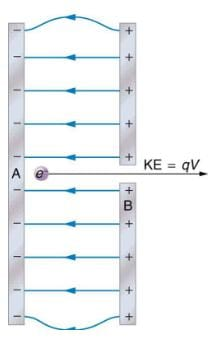On the submicroscopic scale, it is more convenient to define an energy unit called the electron volt (eV), which is the energy given to a fundamental charge accelerated through a potential difference of 1 V. In equation form,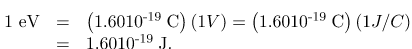Electron Volt:

On the submicroscopic scale, it is more convenient to define an energy unit called the electron volt (eV), which is the energy given to a fundamental charge accelerated through a potential difference of 1 V. In equation form,An electron accelerated through a potential difference of 1 V is given an energy of 1 eV. It follows that an electron accelerated through 50 V is given 50 eV. A potential difference of 100,000 V (100 kV) will give an electron an energy of 100,000 eV (100 keV), and so on. Similarly, an ion with a double positive charge accelerated through 100 V will be given 200 eV of energy. These simple relationships between accelerating voltage and particle charges make the electron volt a simple and convenient energy unit in such circumstances.

Connections: Energy Units
The electron volt (eV) is the most common energy unit for submicroscopic processes. This will be particularly noticeable in the chapters on modern physics. Energy is so important to so many subjects that there is a tendency to define a special energy unit for each major topic. There are, for example, calories for food energy, kilowatt-hours for electrical energy, and therms for natural gas energy.

Conservation of Energy:

The total energy of a system is conserved if there is no net addition (or subtraction) of work or heat transfer. For conservative forces, such as the electrostatic force, conservation of energy states that mechanical energy is a constant.
Mechanical energy is the sum of the kinetic energy and potential energy of a system; that is, KE+PE=constant. A loss of PE of a charged particle becomes an increase in its KE. Here PE is the electric potential energy. Conservation of energy is stated in equation form as

KE+PE=constant
or
KE+ PE= KEf + PEf,
where i and f stand for initial and final conditions. As we have found many times before, considering energy can give us insights and facilitate problem solving.

Electrical Potential Energy Converted to Kinetic Energy

Calculate the final speed of a free electron accelerated from rest through a potential difference of 100 V. (Assume that this numerical value is accurate to three significant figures.)
Strategy
We have a system with only conservative forces. Assuming the electron is accelerated in a vacuum, and neglecting the gravitational force (we will check on this assumption later), all of the electrical potential energy is converted into kinetic energy. We can identify the initial and final forms of energy to be KEi =0, KEf=mv2, PEi=qV, and PEf=0.

Solution

Conservation of energy states that

KE+ PE= KEf + PEf.

Entering the forms identified above, we obtain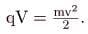We solve this for u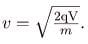Entering values for q,V, and m gives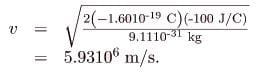Discussion

Note that both the charge and the initial voltage are negative. From the discussions in Electric Charge and Electric Field, we know that electrostatic forces on small particles are generally very large compared with the gravitational force. The large final speed confirms that the gravitational force is indeed negligible here. The large speed also indicates how easy it is to accelerate electrons with small voltages because of their very small mass. Voltages much higher than the 100 V in this problem are typically used in electron guns. Those higher voltages produce electron speeds so great that relativistic effects must be taken into account. That is why a low voltage is considered (accurately) in this example.

Section Summary

• Electric potential is potential energy per unit charge.
• The potential difference between points A and B, VB-VA, defined to be the change in potential energy of a charge q moved from A to B, is equal to the change in potential energy divided by the charge, Potential difference is commonly called voltage, represented by the symbol V.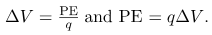• An electron volt is the energy given to a fundamental charge accelerated through a potential difference of 1 V. In equation form,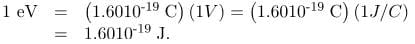• Mechanical energy is the sum of the kinetic energy and potential energy of a system, that is, KE + PE. This sum is a constant.

Conceptual Questions

Voltage is the common word for potential difference. Which term is more descriptive, voltage or potential difference?

If the voltage between two points is zero, can a test charge be moved between them with zero net work being done? Can this necessarily be done without exerting a force? Explain.

What is the relationship between voltage and energy? More precisely, what is the relationship between potential difference and electric potential energy?

Voltages are always measured between two points. Why?

How are units of volts and electron volts related? How do they differ?

Problems & Exercises

Find the ratio of speeds of an electron and a negative hydrogen ion (one having an extra electron) accelerated through the same voltage, assuming non-relativistic final speeds. Take the mass of the hydrogen ion to be 1.6710-27 kg.
42.8
An evacuated tube uses an accelerating voltage of 40 kV to accelerate electrons to hit a copper plate and produce x rays.   Non-relativistically, what would be the maximum speed of these electrons?
A bare helium nucleus has two positive charges and a mass of 1.6710-27 kg. (a) Calculate its kinetic energy in joules at 2.00% of the speed of light. (b) What is this in electron volts? (c) What voltage would be needed to obtain this energy?

Integrated Concepts

Singly charged gas ions are accelerated from rest through a voltage of 13.0 V. At what temperature will the average kinetic energy of gas molecules be the same as that given these ions?
1.00105 K

Integrated Concepts

The temperature near the center of the Sun is thought to be 15 million degrees Celsius (1.5107 C). Through what voltage must a singly charged ion be accelerated to have the same energy as the average kinetic energy of ions at this temperature?

Integrated Concepts

(a) What is the average power output of a heart defibrillator that dissipates 400 J of energy in 10.0 ms? (b) Considering the high-power output, why doesn’t the defibrillator produce serious burns?
(a) 4104 W
(b) A defibrillator does not cause serious burns because the skin conducts electricity well at high voltages, like those used in defibrillators. The gel used aids in the transfer of energy to the body, and the skin doesn’t absorb the energy, but rather lets it pass through to the heart.

Integrated Concepts

A lightning bolt strikes a tree, moving 20.0 C of charge through a potential difference of 1.00102 MV. (a) What energy was dissipated? (b) What mass of water could be raised from 15C to the boiling point and then boiled by this energy? (c) Discuss the damage that could be caused to the tree by the expansion of the boiling steam.

Integrated Concepts

A 12.0 V battery-operated bottle warmer heats 50.0 g of glass, 2.5102 g of baby formula, and 2.00102 g of aluminum from 20.0C to 90.0C. (a) How much charge is moved by the battery? (b) How many electrons per second flow if it takes 5.00 min to warm the formula? (Hint: Assume that the specific heat of baby formula is about the same as the specific heat of water.)
(a) 7.40103 C
(b) 1.541020 electrons per second.

Integrated Concepts

A battery-operated car utilizes a 12.0 V system. Find the charge the batteries must be able to move in order to accelerate the 750 kg car from rest to 25.0 m/s, make it climb a 2.00202 m high hill, and then cause it to travel at a constant 25.0 m/s by exerting a 5.00102 N force for an hour.
3.89106

Integrated Concepts

Fusion probability is greatly enhanced when appropriate nuclei are brought close together, but mutual Coulomb repulsion must be overcome. This can be done using the kinetic energy of high-temperature gas ions or by accelerating the nuclei toward one another. (a) Calculate the potential energy of two singly charged nuclei separated by 1.0010-12 m by finding the voltage of one at that distance and multiplying by the charge of the other. (b) At what temperature will atoms of a gas have an average kinetic energy equal to this needed electrical potential energy?

Unreasonable Results

(a) Find the voltage near a 10.0 cm diameter metal sphere that has 8.00 C of excess positive charge on it. (b) What is unreasonable about this result? (c) Which assumptions are responsible?

(a) 1.441012 V

(b) This voltage is very high. A 10.0 cm diameter sphere could never maintain this voltage; it would discharge.

(c) An 8.00 C charge is more charge than can reasonably be accumulated on a sphere of that size.

Consider a battery used to supply energy to a cellular phone. Construct a problem in which you determine the energy that must be supplied by the battery, and then calculate the amount of charge it must be able to move in order to supply this energy. Among the things to be considered are the energy needs and battery voltage. You may need to look ahead to interpret manufacturer’s battery ratings in ampere-hours as energy in joules.

The document Electric Potential Energy: Potential Difference Notes | Study Basic Physics for IIT JAM - Physics is a part of the Physics Course Basic Physics for IIT JAM.
All you need of Physics at this link: PhysicsUse Code STAYHOME200 and get INR 200 additional OFF

## Basic Physics for IIT JAM

220 videos|156 docs|94 tests

Track your progress, build streaks, highlight & save important lessons and more!

,

,

,

,

,

,

,

,

,

,

,

,

,

,

,

,

,

,

,

,

,

;